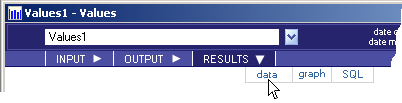Values - RESULTS - Data - Teradata Warehouse Miner - 5.4.4

Teradata Warehouse Miner User Guide - Volume 1Introduction and Profiling

prodname
vrm_release
5.4.4
category
User Guide
featnum
B035-2300-077K
1. On the Values dialog box, click on RESULTS.
2. Click on data.
Values > Results > DataResults data, if any, is displayed in a data grid as described in RESULTS Tab.

The following is a description of the results returned by the analysis, depending on the options selected. If an output table is created, the columns in bold below comprise the Unique Primary Index (UPI) of the output table.

Data Results for Values
Name Type Definition
COL Group By Column Type Column(s) will be created only if the “group” parameter is specified. Multiple columns, if specified, will be created or displayed as the column name. These column(s) will contain the unique values that the group by column takes on.
xtbl VARCHAR (30) Table that the variable for the values operation resides in, as specified by the “table” parameter.
xcol VARCHAR (30) Variable that the values operation will be run against, as specified by the “column” parameter.
xtype VARCHAR (30) The data type of this variable.
xcnt FLOAT The total number of occurrences of this variable.
xnull FLOAT Total number of rows where this variable takes on a null value.
xunique FLOAT Total number of rows where this variable takes on a unique value.
xblank FLOAT Total number of rows where this variable is blank.
xzero FLOAT Total number of rows where this variable is equal to 0.
xpos FLOAT Total number of rows where this variable has a positive value.
xneg FLOAT Total number of rows where this variable has a negative value.